##### Physics I For Dummies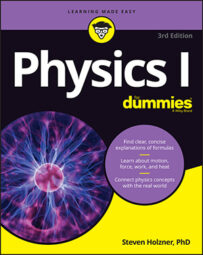In space, gravity supplies the centripetal force that causes satellites (like the moon) to orbit larger bodies (like the Earth). Thanks to physics, if you know the mass and altitude of a satellite in orbit around the Earth, you can calculate how quickly it needs to travel to maintain that orbit.

A particular satellite can have only one speed when in orbit around a particular body at a given distance because the force of gravity doesn’t change. So what’s that speed? You can calculate it with the equations for centripetal force and gravitational force. For a satellite of a particular mass, m1, to orbit, you need a corresponding centripetal force: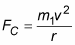This centripetal force has to come from the force of gravity, so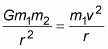You can rearrange this equation to get the speed: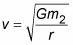This equation represents the speed that a satellite at a given radius must travel in order to orbit if the orbit is due to gravity. The speed can’t vary as long as the satellite has a constant orbital radius — that is, as long as it’s going around in circles. This equation holds for any orbiting object where the attraction is the force of gravity, whether it’s a human-made satellite orbiting the Earth or the Earth orbiting the sun. If you want to find the speed for satellites that orbit the Earth, for example, you use the mass of the Earth in the equation: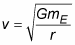Here are a few details you should note on reviewing the orbiting speed equation:

• You have to use the distance from the center of the Earth, not the distance above Earth’s surface, as the radius. Therefore, the distance you use in the equation is the distance between the two orbiting bodies. In this case, you add the distance from the center of the Earth to the surface of the Earth, 6.38 × 106 meters, to the satellite’s height above the Earth.

• The equation assumes that the satellite is high enough off the ground that it orbits out of the atmosphere. That assumption isn’t really true for artificial satellites; even at 400 miles above the surface of the Earth, satellites do feel air friction. Gradually, the drag of friction brings them lower and lower, and when they hit the atmosphere, they burn up on re-entry. When a satellite is less than 100 miles above the surface, its orbit decays appreciably each time it circles the Earth. (Look out below!)

• The equation is independent of mass. If the moon rather than the artificial satellite orbited at 400 miles and you could ignore air friction and collisions with the Earth, it would have to go at the same speed as the satellite in order to preserve its close orbit (which would make for some pretty spectacular moonrises).

Human-made satellites typically orbit at heights of 400 miles from the surface of the Earth (about 640 kilometers, or 6.4 × 105 meters). What’s the speed of such a satellite? All you have to do is put in the numbers: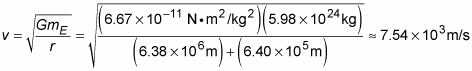This converts to about 16,800 miles per hour.